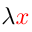Home Higher-Order Logic ExplorerTheorem List (p. 3 of 3) < Previous  Wrap > Bad symbols? Try the GIF version. Mirrors  >  Metamath Home Page  >  HOLE Home Page  >  Theorem List Contents       This page: Page List

Theorem List for Higher-Order Logic Explorer - 201-222   *Has distinct variable group(s)
TypeLabelDescription
Statement

Theoremexnal 201* Theorem 19.14 of [Margaris] p. 90. (Contributed by Mario Carneiro, 10-Oct-2014.)
A:∗       ⊤⊧[(λx:αA)) = (¬ (λx:α A))]

Axiomax-inf 202 The axiom of infinity: the set of "individuals" is not Dedekind-finite. Using the axiom of choice, we can show that this is equivalent to an embedding of the natural numbers in ι. (Contributed by Mario Carneiro, 10-Oct-2014.)
⊤⊧(λf:(ιι) [(1-1 f:(ιι)) (¬ (onto f:(ιι)))])

0.6  Rederive the Metamath axioms

Theoremax1 203 Axiom Simp. Axiom A1 of [Margaris] p. 49. (Contributed by Mario Carneiro, 9-Oct-2014.)
R:∗    &   S:∗       ⊤⊧[R ⇒ [SR]]

Theoremax2 204 Axiom Frege. Axiom A2 of [Margaris] p. 49. (Contributed by Mario Carneiro, 9-Oct-2014.)
R:∗    &   S:∗    &   T:∗       ⊤⊧[[R ⇒ [ST]] ⇒ [[RS] ⇒ [RT]]]

Theoremax3 205 Axiom Transp. Axiom A3 of [Margaris] p. 49. (Contributed by Mario Carneiro, 10-Oct-2014.)
R:∗    &   S:∗       ⊤⊧[[(¬ R) ⇒ (¬ S)] ⇒ [SR]]

Theoremaxmp 206 Rule of Modus Ponens. The postulated inference rule of propositional calculus. See e.g. Rule 1 of [Hamilton] p. 73. (Contributed by Mario Carneiro, 10-Oct-2014.)
S:∗    &   ⊤⊧R    &   ⊤⊧[RS]       ⊤⊧S

Theoremax5 207* Axiom of Quantified Implication. Axiom C4 of [Monk2] p. 105. (Contributed by Mario Carneiro, 10-Oct-2014.)
R:∗    &   S:∗       ⊤⊧[(λx:α [RS]) ⇒ [(λx:α R) ⇒ (λx:α S)]]

Theoremax6 208* Axiom of Quantified Negation. Axiom C5-2 of [Monk2] p. 113. (Contributed by Mario Carneiro, 10-Oct-2014.)
R:∗       ⊤⊧[(¬ (λx:α R)) ⇒ (λx:α (¬ (λx:α R)))]

Theoremax7 209* Axiom of Quantifier Commutation. Axiom scheme C6' in [Megill] p. 448 (p. 16 of the preprint). (Contributed by Mario Carneiro, 10-Oct-2014.)
R:∗       ⊤⊧[(λx:α (λy:α R)) ⇒ (λy:α (λx:α R))]

Theoremaxgen 210* Rule of Generalization. See e.g. Rule 2 of [Hamilton] p. 74. (Contributed by Mario Carneiro, 10-Oct-2014.)
⊤⊧R       ⊤⊧(λx:α R)

Theoremax8 211 Axiom of Equality. Axiom scheme C8' in [Megill] p. 448 (p. 16 of the preprint). Also appears as Axiom C7 of [Monk2] p. 105. (Contributed by Mario Carneiro, 10-Oct-2014.)
A:α    &   B:α    &   C:α       ⊤⊧[[A = B] ⇒ [[A = C] ⇒ [B = C]]]

Theoremax9 212* Axiom of Equality. Axiom scheme C8' in [Megill] p. 448 (p. 16 of the preprint). Also appears as Axiom C7 of [Monk2] p. 105. (Contributed by Mario Carneiro, 10-Oct-2014.)
A:α       ⊤⊧(¬ (λx:α (¬ [x:α = A])))

Theoremax10 213* Axiom of Quantifier Substitution. Appears as Lemma L12 in [Megill] p. 445 (p. 12 of the preprint). (Contributed by Mario Carneiro, 10-Oct-2014.)
⊤⊧[(λx:α [x:α = y:α]) ⇒ (λy:α [y:α = x:α])]

Theoremax11 214* Axiom of Variable Substitution. It is based on Lemma 16 of [Tarski] p. 70 and Axiom C8 of [Monk2] p. 105, from which it can be proved by cases. (Contributed by Mario Carneiro, 10-Oct-2014.)
A:∗       ⊤⊧[[x:α = y:α] ⇒ [(λy:α A) ⇒ (λx:α [[x:α = y:α] ⇒ A])]]

Theoremax12 215* Axiom of Quantifier Introduction. Axiom scheme C9' in [Megill] p. 448 (p. 16 of the preprint). (Contributed by Mario Carneiro, 10-Oct-2014.)
⊤⊧[(¬ (λz:α [z:α = x:α])) ⇒ [(¬ (λz:α [z:α = y:α])) ⇒ [[x:α = y:α] ⇒ (λz:α [x:α = y:α])]]]

Theoremax13 216 Axiom of Equality. Axiom scheme C12' in [Megill] p. 448 (p. 16 of the preprint). It is a special case of Axiom B8 (p. 75) of system S2 of [Tarski] p. 77. (Contributed by Mario Carneiro, 10-Oct-2014.)
A:α    &   B:α    &   C:(α → ∗)       ⊤⊧[[A = B] ⇒ [(CA) ⇒ (CB)]]

Theoremax14 217 Axiom of Equality. Axiom scheme C12' in [Megill] p. 448 (p. 16 of the preprint). It is a special case of Axiom B8 (p. 75) of system S2 of [Tarski] p. 77. (Contributed by Mario Carneiro, 10-Oct-2014.)
A:(α → ∗)    &   B:(α → ∗)    &   C:α       ⊤⊧[[A = B] ⇒ [(AC) ⇒ (BC)]]

Theoremax17m 218* Axiom to quantify a variable over a formula in which it does not occur. Axiom C5 in [Megill] p. 444 (p. 11 of the preprint). Also appears as Axiom B6 (p. 75) of system S2 of [Tarski] p. 77 and Axiom C5-1 of [Monk2] p. 113. (Contributed by Mario Carneiro, 10-Oct-2014.)
A:∗       ⊤⊧[A ⇒ (λx:α A)]

Theoremaxext 219* Axiom of Extensionality. An axiom of Zermelo-Fraenkel set theory. It states that two sets are identical if they contain the same elements. Axiom Ext of [BellMachover] p. 461. (Contributed by Mario Carneiro, 10-Oct-2014.)
A:(α → ∗)    &   B:(α → ∗)       ⊤⊧[(λx:α [(Ax:α) = (Bx:α)]) ⇒ [A = B]]

Theoremaxrep 220* Axiom of Replacement. An axiom scheme of Zermelo-Fraenkel set theory. Axiom 5 of [TakeutiZaring] p. 19. (Contributed by Mario Carneiro, 10-Oct-2014.)
A:∗    &   B:(α → ∗)       ⊤⊧[(λx:α (λy:β (λz:β [(λy:β A) ⇒ [z:β = y:β]]))) ⇒ (λy:(β → ∗) (λz:β [(y:(β → ∗)z:β) = (λx:α [(Bx:α) (λy:β A)])]))]

Theoremaxpow 221* Axiom of Power Sets. An axiom of Zermelo-Fraenkel set theory. (Contributed by Mario Carneiro, 10-Oct-2014.)
A:(α → ∗)       ⊤⊧(λy:((α → ∗) → ∗) (λz:(α → ∗) [(λx:α [(z:(α → ∗)x:α) ⇒ (Ax:α)]) ⇒ (y:((α → ∗) → ∗)z:(α → ∗))]))

Theoremaxun 222* Axiom of Union. An axiom of Zermelo-Fraenkel set theory. (Contributed by Mario Carneiro, 10-Oct-2014.)
A:((α → ∗) → ∗)       ⊤⊧(λy:(α → ∗) (λz:α [(λx:(α → ∗) [(x:(α → ∗)z:α) (Ax:(α → ∗))]) ⇒ (y:(α → ∗)z:α)]))

Page List